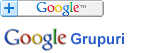### Căutare în Webster - Dicționarul explicativ al limbii engleze

 Cuvânt
Pentru căutare rapidă introduceți minim 3 litere.

## EQUATION - Definiția din dicționar

Notă: Puteţi căuta fiecare cuvânt din cadrul definiţiei printr-un simplu click pe cuvântul dorit.

E*qua"tion (?), n. [L. aequatio an equalizing: cf. F. équation equation. See Equate.] 1.
[1913 Webster]

Again the golden day resumed its right,
And ruled in just equation with the night.
Rowe.
[1913 Webster]

2. (Math.)
[1913 Webster]

3. (Astron.)
[1913 Webster]

Absolute equation. See under Absolute. -- Equation box, or Equational box, a system of differential gearing used in spinning machines for regulating the twist of the yarn. It resembles gearing used in equation clocks for showing apparent time. -- Equation of the center (Astron.), the difference between the place of a planet as supposed to move uniformly in a circle, and its place as moving in an ellipse. -- Equations of condition (Math.), equations formed for deducing the true values of certain quantities from others on which they depend, when different sets of the latter, as given by observation, would yield different values of the quantities sought, and the number of equations that may be found is greater than the number of unknown quantities. -- Equation of a curve (Math.), an equation which expresses the relation between the co&ö;rdinates of every point in the curve. -- Equation of equinoxes (Astron.), the difference between the mean and apparent places of the equinox. -- Equation of payments (Arith.), the process of finding the mean time of payment of several sums due at different times. -- Equation of time (Astron.), the difference between mean and apparent time, or between the time of day indicated by the sun, and that by a perfect clock going uniformly all the year round. -- Equation clock or Equation watch, a timepiece made to exhibit the differences between mean solar and apparent solar time. Knight. -- Normal equation. See under Normal. -- Personal equation (Astron.), the difference between an observed result and the true qualities or peculiarities in the observer; particularly the difference, in an average of a large number of observation, between the instant when an observer notes a phenomenon, as the transit of a star, and the assumed instant of its actual occurrence; or, relatively, the difference between these instants as noted by two observers. It is usually only a fraction of a second; -- sometimes applied loosely to differences of judgment or method occasioned by temperamental qualities of individuals. -- Theory of equations (Math.), the branch of algebra that treats of the properties of a single algebraic equation of any degree containing one unknown quantity.
[1913 Webster]